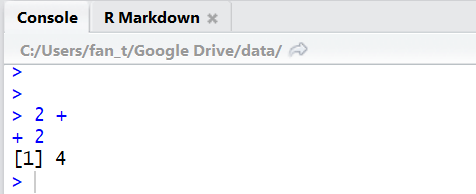# R Tutorial 3: Basic Programming

## How to write code

R commands can be either written onto a R script or directly into the console pane. In the R script, you can either press the run button on top, or Ctrl+Enter for Windows, and Command+Enter. Let’s do some quick math 😉

In R Script:

```2+2
Output:  4```
```4-1
Output:  3```
```100:120
Output:  100 101 102 103 104 105 106 107 108 109 110 111 112 113 114 115 116 ##  117 118 119 120 #returns numbers from 100 to 120 in a vector (which we will be covering later)```

In Console Pane:R is a dynamic programming language, hence you can type an incomplete command, and R will prompt you to complete the rest of the command. Either finish the command or hit esc to start over.

## Creating R Objects

R works on things called objects, or variables. You can save a number, a name, or even a table of 35 thousand rows of data into a R object.

Some rules for naming R Objects:

• Must start with a letter (a-z)
• Case Sensitive (Name and name is different objects)
• Cannot use some special symbols (like ,!,\$,@ as they are used for some other operations)
GoodNames BadNames
a 1trial
b \$
FOO mean
my_var 2nd
day.2 !bad

There are three main approach in creating R Object:

• Approach One: a<-1
• Approach Two: a = 1
• Approach Three: assign(“a”,1)

Once created, the object will appear in the environment pane, with some basic information about the data type. It will stay there once it is created, so you might want to clear the environment now and then with the broomstick to remove some clutter after some amount of work as seen below## Math Operators

Given that a = 2 and b = 3:

Operator Syntax Results
+ a+b 5
a-b -1
* a*b 6
/ a/b 0.6666667
^ or ** ab or a**b 8
%% a%%b 2
%/% a%/%b 0

##Logical Operators

Operator Syntax Meaning
> a>b Is a greater than b?
>= a>=b Is a greater than or equal to b
< a<b Is a less than b
<= a<=b Is a less than or equal to be
== a==b Is a equal to b?
!= a!=b Is a not equal to b?
%in a %in% c(a,b,c) Is a in the group a,b,c?
& a&b Is both a and b true?
| a|b Is either a or b true?

Doing comparison of objects with R with operators return “True” or “False” values, which is useful for if statements and also for loops.

Be careful not to confuse “=” with “==”. Remember “=” is used for assignment of values to objects! This is 90% of my error when I started off coding R @.@

## If/Else Statements

These two statements are core to any programming language, for conditional actions. P.S cat() function is the output functions. You can print variables, vectors and characters using this function

```x = 5
if(x>0){cat("Positive Number")}
else{cat("Negative Number")}```
```Output:
Positive Number```

Next: Tutorial 4 Data Structures

## One thought on “R Tutorial 3: Basic Programming”

1.Sharon says:

In the If/else cat for positive number should it be If(x>0){cat(“Positive Number”)} is the front of the Cat() is missing?

Like